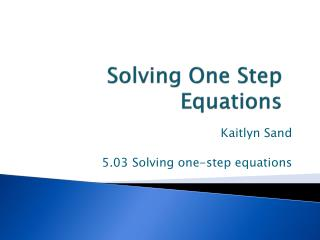DownloadDownload PresentationSolving One Step Equations

# Solving One Step Equations

Download Presentation## Solving One Step Equations

- - - - - - - - - - - - - - - - - - - - - - - - - - - E N D - - - - - - - - - - - - - - - - - - - - - - - - - - -
##### Presentation Transcript

1. Solving One Step Equations Kaitlyn Sand 5.03 Solving one-step equations

2. Order of Operations PEMDAS right to left to solve: (multiplication) (subtraction) (addition)

3. Solving One Step Equations Opposite of PEMDAS; SADMEP. • S – subtraction • A – addition • D – division • M – multiplication • E – exponents (remember, we haven’t • P – parenthesis talked about these yet!)

4. One Step Equations -Perform 1 step to solve -Try to get “X” by itself -Do the same operation to both sides -Just like what you did on excel math:

5. Excel Math + 3 = 5 Now, we use X as a place holder instead of the empty square. X + 3 = 5

6. What you do to ONE side, you MUST do to the other. X + 3 = 5 (Subtract 3 from both sides) -3 -3 X = 2

7. 2X = 10 2 2 X = 5 6 – 1X = 2 -6 -6 -1X = -4 -1 -1 X = 4

8. Foamy Squishy Things Introduction = X + = 0 = 1 = -1

9. X + 3 = 5 You can also use foamy squishy things to solve problems. += =

10. Using Foamy Squishy Things Set up your foamy squishy things in the form of this problem: X + ˉ5 = 2